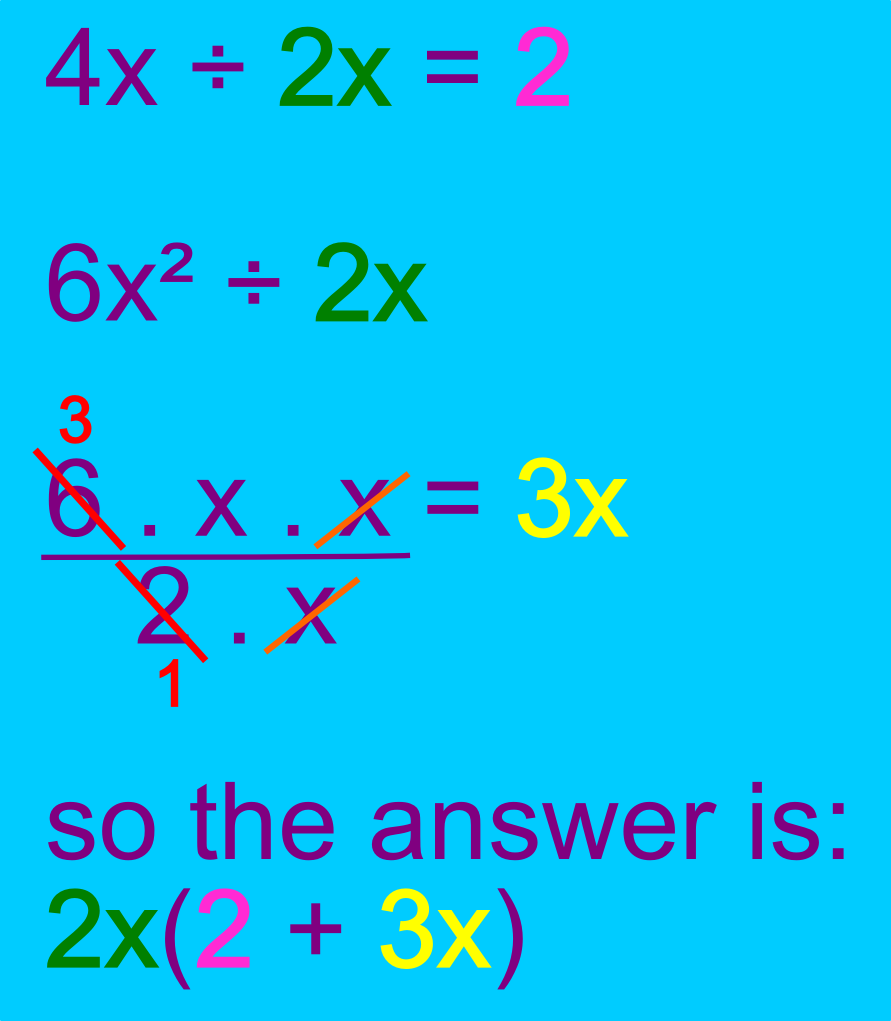The Singing Hedgehog Guide to:
Algebra - Factorisation 2

Here is a simple example:
Factorise 4x + 6x²

First find the HCF "numbers then letters"
We can divide 4 and 6 by 2
We can divide x and x² by x
The HCF is simply 2x

Now divide each term by the
HCFWe can always check by mulitiplying back out:
2x.2 + 2x.3x = 4x + 6x²

next page